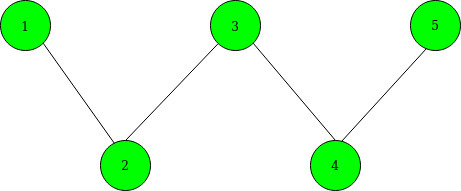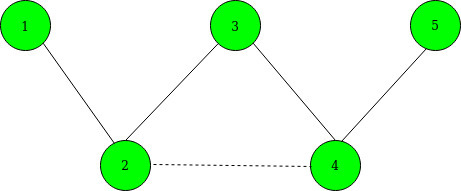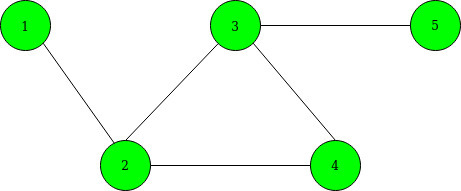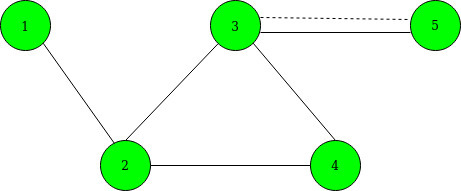GFG App
Open AppBrowser
Continue

# Maximize shortest path between given vertices by adding a single edge

Given an undirected graph of N nodes and M vertices. You are also given a K edges as selected[]. The task to maximize the shortest path length between node 1 to node N by adding single edges between any two vertices from the given selected edges.
Note: You may add an edge between any two selected vertices who have already an edge between them.

Input: N = 5, M = 4, K = 2, selected[] = {2, 4}
Below is the given graph:Output:
Explanation:
Before adding an edge between 2 and 4, the Shortest Path becomes: 1–>2–>3–>4–>5.
After adding an edge between 2 and 4, the Shortest Path becomes 1–>2–>4–>5. Below is the graph after adding edges. denoted by the dashed line.Input: N = 5 M = 5 K = 3 selected[] = {1, 3, 5}
Below is the given graph:Output:
Explanation:
We can add an edge between 3 and 5 as they have already an edge between them. so, the shortest path becomes 1–>2–>3–>5. Below is the graph after adding edges. denoted by the dashed line.Approach: The idea is to use Breadth-First Search to find the distance from vertices 1 and N to each selected vertex. For selected vertex i, let xi denote the distance to node 1, and yi denote the distance to node N. Below are the steps:

1. Maintain a 2D matrix(say dist[]) having 2 rows and N columns.
2. In the first row, Maintain the shortest distance between node 1 and other vertices in the graph using BFS Traversal.
3. In the second row, Maintain the shortest distance between node N and the other vertices of the graph using BFS Traversal.
4. Now, choose two selected vertices a and b from selected[] to minimize the value of min(xa + yb, ya + xb). For this do the following:
• Create a vector of pairs and store the value of (xi – yi) with their respective selected node.
• Sort the above vector of pairs.
• Initialize best to 0 and max to -INF.
• Now traverse the above vector of pairs and for each selected node(say a) update the value of best to maximum of (best, max + dist[a]) and update max to maximum of (max, dist[a]).
5. After the above operations the maximum of (dist[N-1] and best + 1) given the minimum shortest path.

Below is the implementation of the above approach:

## C++

 `// C++ program for the above approach` `#include ` `using` `namespace` `std;` `const` `int` `INF = 1e9 + 7;` `int` `N, M;`   `// To store graph as adjacency list` `vector<``int``> edges;`   `// To store the shortest path` `int` `dist;`   `// Function that performs BFS Traversal` `void` `bfs(``int``* dist, ``int` `s)` `{` `    ``int` `q;`   `    ``// Fill initially each distance as INF` `    ``fill(dist, dist + N, INF);` `    ``int` `qh = 0, qt = 0;` `    ``q[qh++] = s;` `    ``dist[s] = 0;`   `    ``// Perform BFS` `    ``while` `(qt < qh) {`   `        ``int` `x = q[qt++];`   `        ``// Traverse the current edges` `        ``for` `(``int` `y : edges[x]) {` `            ``if` `(dist[y] == INF) {`   `                ``// Update the distance` `                ``dist[y] = dist[x] + 1;`   `                ``// Insert in queue` `                ``q[qh++] = y;` `            ``}` `        ``}` `    ``}` `}`   `// Function that maximizes the shortest` `// path between source and destination` `// vertex by adding a single edge between` `// given selected nodes` `void` `shortestPathCost(``int` `selected[], ``int` `K)` `{` `    ``vector > data;`   `    ``// To update the shortest distance` `    ``// between node 1 to other vertices` `    ``bfs(dist, 0);`   `    ``// To update the shortest distance` `    ``// between node N to other vertices` `    ``bfs(dist, N - 1);`   `    ``for` `(``int` `i = 0; i < K; i++) {`   `        ``// Store the values x[i] - y[i]` `        ``data.emplace_back(dist[selected[i]]` `                              ``- dist[selected[i]],` `                          ``selected[i]);` `    ``}`   `    ``// Sort all the vectors of pairs` `    ``sort(data.begin(), data.end());` `    ``int` `best = 0;` `    ``int` `MAX = -INF;`   `    ``// Traverse data[]` `    ``for` `(``auto` `it : data) {` `        ``int` `a = it.second;` `        ``best = max(best,` `                   ``MAX + dist[a]);`   `        ``// Maximize x[a] - y[b]` `        ``MAX= max(MAX, dist[a]);` `    ``}`   `    ``// Print minimum cost` `    ``printf``(``"%d\n"``, min(dist[N - 1], best + 1));` `}`   `// Driver Code` `int` `main()` `{` `    ``// Given nodes and edges` `    ``N = 5, M = 4;` `    ``int` `K = 2;` `    ``int` `selected[] = { 1, 3 };`   `    ``// Sort the selected nodes` `    ``sort(selected, selected + K);`   `    ``// Given edges` `    ``edges.push_back(1);` `    ``edges.push_back(0);` `    ``edges.push_back(2);` `    ``edges.push_back(1);` `    ``edges.push_back(3);` `    ``edges.push_back(2);` `    ``edges.push_back(4);` `    ``edges.push_back(3);`   `    ``// Function Call` `    ``shortestPathCost(selected, K);` `    ``return` `0;` `}`

## Java

 `// Java program for the above approach` `import` `java.util.*;` `import` `java.lang.*;`   `class` `GFG{` `    `  `static` `int` `INF = (``int``)1e9 + ``7``; ` `static` `int` `N, M; ` `  `  `// To store graph as adjacency list ` `static` `ArrayList> edges; ` `  `  `// To store the shortest path ` `static` `int``[][] dist = ``new` `int``[``2``][``200000``]; ` `  `  `// Function that performs BFS Traversal ` `static` `void` `bfs(``int``[] dist, ``int` `s) ` `{ ` `    ``int``[] q = ``new` `int``[``200000``]; ` `  `  `    ``// Fill initially each distance as INF ` `    ``Arrays.fill(dist, INF); ` `    `  `    ``int` `qh = ``0``, qt = ``0``; ` `    ``q[qh++] = s; ` `    ``dist[s] = ``0``; ` `  `  `    ``// Perform BFS ` `    ``while` `(qt < qh) ` `    ``{ ` `        ``int` `x = q[qt++]; ` `  `  `        ``// Traverse the current edges ` `        ``for``(Integer y : edges.get(x))` `        ``{ ` `            ``if` `(dist[y] == INF) ` `            ``{ ` `                `  `                ``// Update the distance ` `                ``dist[y] = dist[x] + ``1``; ` `  `  `                ``// Insert in queue ` `                ``q[qh++] = y; ` `            ``} ` `        ``} ` `    ``} ` `} ` `  `  `// Function that maximizes the shortest ` `// path between source and destination ` `// vertex by adding a single edge between ` `// given selected nodes ` `static` `void` `shortestPathCost(``int` `selected[], ``int` `K) ` `{ ` `    ``ArrayList<``int``[]> data = ``new` `ArrayList<>(); ` `  `  `    ``// To update the shortest distance ` `    ``// between node 1 to other vertices ` `    ``bfs(dist[``0``], ``0``); ` `  `  `    ``// To update the shortest distance ` `    ``// between node N to other vertices ` `    ``bfs(dist[``1``], N - ``1``); ` `  `  `    ``for``(``int` `i = ``0``; i < K; i++) ` `    ``{ ` `        `  `        ``// Store the values x[i] - y[i] ` `        ``data.add(``new` `int``[]{dist[``0``][selected[i]] - ` `                           ``dist[``1``][selected[i]], ` `                                   ``selected[i]}); ` `    ``} ` `  `  `    ``// Sort all the vectors of pairs ` `    ``Collections.sort(data, (a, b) -> a[``0``] - b[``0``]); ` `    ``int` `best = ``0``; ` `    ``int` `MAX = -INF; ` `  `  `    ``// Traverse data[] ` `    ``for``(``int``[] it : data) ` `    ``{ ` `        ``int` `a = it[``1``]; ` `        ``best = Math.max(best, ` `                        ``MAX + dist[``1``][a]); ` `  `  `        ``// Maximize x[a] - y[b] ` `        ``MAX = Math.max(MAX, dist[``0``][a]); ` `    ``} ` `    `  `    ``// Print minimum cost ` `    ``System.out.println(Math.min(dist[``0``][N - ``1``],` `                                     ``best + ``1``)); ` `}`   `// Driver code` `public` `static` `void` `main (String[] args)` `{` `    `  `    ``// Given nodes and edges ` `    ``N = ``5``; M = ``4``; ` `    ``int` `K = ``2``; ` `    ``int` `selected[] = { ``1``, ``3` `}; ` `    `  `    ``// Sort the selected nodes ` `    ``Arrays.sort(selected);` `    `  `    ``edges = ``new` `ArrayList<>();` `    `  `    ``for``(``int` `i = ``0``; i < ``200005``; i++)` `        ``edges.add(``new` `ArrayList());` `    `  `    ``// Given edges ` `    ``edges.get(``0``).add(``1``); ` `    ``edges.get(``1``).add(``0``); ` `    ``edges.get(``1``).add(``2``); ` `    ``edges.get(``2``).add(``1``); ` `    ``edges.get(``2``).add(``3``); ` `    ``edges.get(``3``).add(``2``); ` `    ``edges.get(``3``).add(``4``); ` `    ``edges.get(``4``).add(``3``); ` `    `  `    ``// Function Call ` `    ``shortestPathCost(selected, K); ` `}` `}`   `// This code is contributed by offbeat`

## Python3

 `# Python3 program for the above approach`   `# Function that performs BFS Traversal` `def` `bfs(x, s):` `    ``global` `edges, dist` `    ``q ``=` `[``0` `for` `i ``in` `range``(``200000``)]`   `    ``# Fill initially each distance as INF` `    ``# fill(dist, dist + N, INF)` `    ``qh, qt ``=` `0``, ``0` `    ``q[qh] ``=` `s` `    ``qh ``+``=` `1` `    ``dist[x][s] ``=` `0`   `    ``# Perform BFS` `    ``while` `(qt < qh):` `        ``xx ``=` `q[qt]` `        ``qt ``+``=` `1`   `        ``# Traverse the current edges` `        ``for` `y ``in` `edges[xx]:` `            ``if` `(dist[x][y] ``=``=` `10``*``*``18``):`   `                ``# Update the distance` `                ``dist[x][y] ``=` `dist[x][xx] ``+` `1`   `                ``# Insert in queue` `                ``q[qh] ``=` `y` `                ``qh ``+``=` `1`   `# Function that maximizes the shortest` `# path between source and destination` `# vertex by adding a single edge between` `# given selected nodes` `def` `shortestPathCost(selected, K):` `    ``global` `dist, edges` `    ``data ``=` `[]`   `    ``# To update the shortest distance` `    ``# between node 1 to other vertices` `    ``bfs(``0``, ``0``)`   `    ``# To update the shortest distance` `    ``# between node N to other vertices` `    ``bfs(``1``, N ``-` `1``)` `    ``for` `i ``in` `range``(K):`   `        ``# Store the values x[i] - y[i]` `        ``data.append([dist[``0``][selected[i]]``-` `dist[``1``][selected[i]], selected[i]])`   `    ``# Sort all the vectors of pairs` `    ``data ``=` `sorted``(data)` `    ``best ``=` `0` `    ``MAX` `=` `-``10``*``*``18`   `    ``# Traverse data[]` `    ``for` `it ``in` `data:` `        ``a ``=` `it[``1``]` `        ``best ``=` `max``(best,``MAX` `+` `dist[``1``][a])`   `        ``# Maximize x[a] - y[b]` `        ``MAX``=` `max``(``MAX``, dist[``0``][a])`   `    ``# Print minimum cost` `    ``print``(``min``(dist[``0``][N ``-` `1``], best ``+` `1``))`   `# Driver Code` `if` `__name__ ``=``=` `'__main__'``:`   `    ``# Given nodes and edges` `    ``edges ``=` `[[] ``for` `i ``in` `range``(``5``)]` `    ``dist ``=` `[[``10``*``*``18` `for` `i ``in` `range``(``1000005``)] ``for` `i ``in` `range``(``2``)]` `    ``N,M ``=` `5``, ``4` `    ``K ``=` `2` `    ``selected ``=` `[``1``, ``3``]`   `    ``# Sort the selected nodes` `    ``selected ``=` `sorted``(selected)`   `    ``# Given edges` `    ``edges[``0``].append(``1``)` `    ``edges[``1``].append(``0``)` `    ``edges[``1``].append(``2``)` `    ``edges[``2``].append(``1``)` `    ``edges[``2``].append(``3``)` `    ``edges[``3``].append(``2``)` `    ``edges[``3``].append(``4``)` `    ``edges[``4``].append(``3``)`   `    ``# Function Call` `    ``shortestPathCost(selected, K)`   `    ``# This code is contributed by mohit kumar 29`

## C#

 `using` `System;` `using` `System.Linq;`   `class` `Program` `{` `    ``static` `int` `N, M, K;` `    ``static` `int``[][] edges, dist;` `    ``static` `int``[] selected;` `//Function that performs BFS Traversal` `    ``static` `void` `BFS(``int` `x, ``int` `s)` `    ``{` `        ``int``[] q = ``new` `int``;` `        ``//` `    ``//Fill initially each distance as INF` `    ``//fill(dist, dist + N, INF)` `        ``int` `qh = 0, qt = 0;`   `        ``q[qh] = s;` `        ``qh++;` `        ``dist[x][s] = 0;` `//Perform BFS` `        ``while` `(qt < qh)` `        ``{` `            ``int` `xx = q[qt];` `            ``qt++;`   `            ``for` `(``int` `i = 0; i < edges[xx].Length; i++)` `            ``{` `                ``int` `y = edges[xx][i];` `                ``if` `(dist[x][y] == ``int``.MaxValue)` `                ``{` `                    ``dist[x][y] = dist[x][xx] + 1;` `                    ``q[qh] = y;` `                    ``qh++;` `                ``}` `            ``}` `        ``}` `    ``}` `  ``//  Function that maximizes the shortest` `// path between source and destination` `// vertex by adding a single edge between` `// given selected nodes`   `    ``static` `void` `ShortestPathCost(``int``[] selected, ``int` `K)` `    ``{` `        ``BFS(0, 0);` `        ``BFS(1, N - 1);`   `        ``Tuple<``int``, ``int``>[] data = ``new` `Tuple<``int``, ``int``>[K];` `        ``for` `(``int` `i = 0; i < K; i++)` `        ``{` `            ``data[i] = Tuple.Create(dist[selected[i]] - dist[selected[i]], selected[i]);` `        ``}`   `        ``Array.Sort(data, (a, b) => a.Item1.CompareTo(b.Item1));`   `        ``int` `best = 0, MAX = ``int``.MinValue;` `        ``foreach` `(Tuple<``int``, ``int``> it ``in` `data)` `        ``{` `            ``int` `a = it.Item2;` `            ``best = Math.Max(best, MAX + dist[a]);` `            ``MAX = Math.Max(MAX, dist[a]);` `        ``}`   `        ``Console.WriteLine(Math.Min(dist[N - 1], best + 1));` `    ``}` `//Driver code` `    ``static` `void` `Main(``string``[] args)` `    ``{` `        ``N = 5;` `        ``M = 4;` `        ``K = 2;` `        ``selected = ``new` `int``[] { 1, 3 };`   `        ``Array.Sort(selected);`   `        ``edges = ``new` `int``[];` `        ``edges = ``new` `int``[] { 1 };` `        ``edges = ``new` `int``[] { 0, 2 };` `        ``edges = ``new` `int``[] { 1, 3 };` `        ``edges = ``new` `int``[] { 2, 4 };` `        ``edges = ``new` `int``[] { 3 };`   `        ``dist = ``new` `int``[];` `        ``dist = Enumerable.Repeat(``int``.MaxValue, 1000005).ToArray();` `        ``dist = Enumerable.Repeat(``int``.MaxValue, 1000005).ToArray();`   `        ``ShortestPathCost(selected, K);` `    ``}` `}`

## Javascript

 ``

Output:

`3`

Time Complexity: O(N*log N + M)
Auxiliary Space: O(N)

My Personal Notes arrow_drop_up